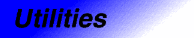# AssignableCategory: utilities Component type: concept

## Description

A type is Assignable if it is possible to copy objects of that type and to assign values to variables.

## Notation

 `X` A type that is a model of Assignable `x`, `y` Object of type `X`

## Valid expressions

NameExpressionType requirementsReturn type
Copy constructor `X(x)`   `X`
Copy constructor
```X x(y);
X x = y;
```

Assignment `x = y`    `X&`
Swap `swap(x,y)`   `void`

## Expression semantics

NameExpressionPreconditionSemanticsPostcondition
Copy constructor `X(x)`     `X(x)` is a copy of `x` 
Copy constructor `X(x)`     `X(x)` is a copy of `x` 
Copy constructor
```X x(y);
X x = y;
```
`x` is a copy of `y` 
Assignment `x` = `y`      `x` is a copy of `y` 
Swap `adobe::swap(x,y)`   Equivalent to
```{
X tmp = x;
x = y;
y = tmp;
}
```

• int